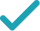Type a math problemFactorSolution Steps
Steps Using Direct Factoring Method
Solution Steps
Factor the expression by grouping. First, the expression needs to be rewritten as . To find and , set up a system to be solved.
Since is positive, and have the same sign. Since is positive, and are both positive. List all such integer pairs that give product .
Calculate the sum for each pair.
The solution is the pair that gives sum .
Rewrite as .
Factor out in the first and in the second group.
Factor out common term by using distributive property.
EvaluateGraph
a+b=11 ab=1\times 24=24
Factor the expression by grouping. First, the expression needs to be rewritten as x^{2}+ax+bx+24. To find a and b, set up a system to be solved.
1,24 2,12 3,8 4,6
Since ab is positive, a and b have the same sign. Since a+b is positive, a and b are both positive. List all such integer pairs that give product 24.
1+24=25 2+12=14 3+8=11 4+6=10
Calculate the sum for each pair.
a=3 b=8
The solution is the pair that gives sum 11.
\left(x^{2}+3x\right)+\left(8x+24\right)
Rewrite x^{2}+11x+24 as \left(x^{2}+3x\right)+\left(8x+24\right).
x\left(x+3\right)+8\left(x+3\right)
Factor out x in the first and 8 in the second group.
\left(x+3\right)\left(x+8\right)
Factor out common term x+3 by using distributive property.
x^{2}+11x+24=0
Quadratic polynomial can be factored using the transformation ax^{2}+bx+c=a\left(x-x_{1}\right)\left(x-x_{2}\right), where x_{1} and x_{2} are the solutions of the quadratic equation ax^{2}+bx+c=0.
x=\frac{-11±\sqrt{11^{2}-4\times 24}}{2}
All equations of the form ax^{2}+bx+c=0 can be solved using the quadratic formula: \frac{-b±\sqrt{b^{2}-4ac}}{2a}. The quadratic formula gives two solutions, one when ± is addition and one when it is subtraction.
x=\frac{-11±\sqrt{121-4\times 24}}{2}
Square 11.
x=\frac{-11±\sqrt{121-96}}{2}
Multiply -4 times 24.
x=\frac{-11±\sqrt{25}}{2}
x=\frac{-11±5}{2}
Take the square root of 25.
x=\frac{-6}{2}
Now solve the equation x=\frac{-11±5}{2} when ± is plus. Add -11 to 5.
x=-3
Divide -6 by 2.
x=\frac{-16}{2}
Now solve the equation x=\frac{-11±5}{2} when ± is minus. Subtract 5 from -11.
x=-8
Divide -16 by 2.
x^{2}+11x+24=\left(x-\left(-3\right)\right)\left(x-\left(-8\right)\right)
Factor the original expression using ax^{2}+bx+c=a\left(x-x_{1}\right)\left(x-x_{2}\right). Substitute -3 for x_{1} and -8 for x_{2}.
x^{2}+11x+24=\left(x+3\right)\left(x+8\right)
Simplify all the expressions of the form p-\left(-q\right) to p+q.
x ^ 2 +11x +24 = 0
Quadratic equations such as this one can be solved by a new direct factoring method that does not require guess work. To use the direct factoring method, the equation must be in the form x^2+Bx+C=0.
r + s = -11 rs = 24
Let r and s be the factors for the quadratic equation such that x^2+Bx+C=(x−r)(x−s) where sum of factors (r+s)=−B and the product of factors rs = C
r = -\frac{11}{2} - u s = -\frac{11}{2} + u
Two numbers r and s sum up to -11 exactly when the average of the two numbers is \frac{1}{2}*-11 = -\frac{11}{2}. You can also see that the midpoint of r and s corresponds to the axis of symmetry of the parabola represented by the quadratic equation y=x^2+Bx+C. The values of r and s are equidistant from the center by an unknown quantity u. Express r and s with respect to variable u. <div style='padding: 8px'><img src='https://opalmath.azureedge.net/customsolver/quadraticgraph.png' style='width: 100%;max-width: 700px' /></div>
(-\frac{11}{2} - u) (-\frac{11}{2} + u) = 24
To solve for unknown quantity u, substitute these in the product equation rs = 24
\frac{121}{4} - u^2 = 24
Simplify by expanding (a -b) (a + b) = a^2 – b^2
-u^2 = 24-\frac{121}{4} = -\frac{25}{4}
Simplify the expression by subtracting \frac{121}{4} on both sides
u^2 = \frac{25}{4} u = \pm\sqrt{\frac{25}{4}} = \pm \frac{5}{2}
Simplify the expression by multiplying -1 on both sides and take the square root to obtain the value of unknown variable u
r =-\frac{11}{2} - \frac{5}{2} = -8 s = -\frac{11}{2} + \frac{5}{2} = -3
The factors r and s are the solutions to the quadratic equation. Substitute the value of u to compute the r and s.